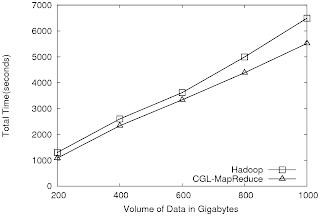## Wednesday, August 20, 2008

### High Energy Physics Data Analysis Using Hadoop and CGL-MapReduce

After the previous set of tests wtih parallel Kmeans clusting using CGL-MapReduce, Hadoop and MPI I shift the direction of testing to another set of tests. This time, the test is to process large number (and volume of) High Energy Physics data files and produce a histogram of interesting events. The amount of data that needs to be processed is 1 terabyte.

Converting this data analysis into a MapReduce version is straigt forward. First the data is split into managable chunks and each map task process some of these chunks and produce histograms of interested events. Reduce tasks merge the resulting histograms producing more concentrated histograms. Finally a merge operation combine all the histograms produced by the reduce tasks.

I performed the above test by incresing the data size on a fixed set of computing nodes using both CGL-MapReduce and Hadoop. To see the scalability of the MapReduce approach and the scalability of the two MapReduce implementations, I performed another test by fixing the amount of data to 100GB and varying the number of compute nodes used. Figure 1 and Figure 2 shows my findings.Figure 1. HEP data analysis, execution time vs. the volume of data (fixed compute resources)

Hadoop and CGL-MapReduce both show similar performance. The amount of data accessed in each analysis is extremely large and hence the performance is limited by the I/O bandwidth of a given node rather than the total processor cores. The overhead induced by the MapReduce implementations has negligible effect on the overall computation.

The results in Figure 2 shows the scalability of the MapReduce technique and the two implementations. It also shows how the performance increase obtained by the parallelism diminshes after a certain number of computation node for this particular data set.